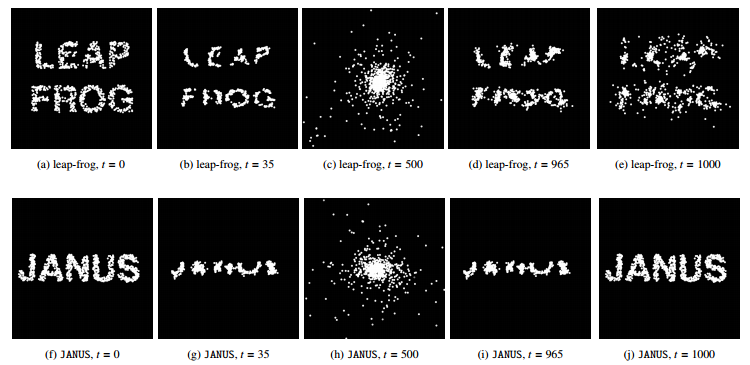# A reversible N-body integrator with JANUS

Authors: Hanno Rein and Daniel Tamayo

First Author’s Institution: Department of Physical and Environmental Sciences, University of Toronto at Scarborough

Status: Submitted to MNRAS, open access

It is simply astronomical, everything in astronomy: there are too many objects, everything happens on mega glacial time scale over mega glacial distance, space is too big, and objects are almost always certainly too faint. With our finite resources and human lifetimes, it is impossible to observe everything and to follow their evolution religiously from the beginning to the end. However, (super)computers have endowed astronomers with divine powers, allowing us to manipulate the Universe at our fingertips. In the absence of data, they are also the next favorite playgrounds to put theories to the test. Simulations have become the bread and butter of astronomical research.

The underlying schemes used in simulations can be divided into those that deal with collisional fluid (hydrodynamics simulations) and those that deal with pure gravitational dynamics (N-body simulation), although there are growing instances of hybrid simulations. The Millennium Simulation is an example of an N-body simulation. In today’s bite, we focus on N-body simulation and discuss a numerical method (aka an integrator) that solves a problem facing all dynamical integrators, which is that of time-reversibility.

If we back-evolve a system under the influence of gravity after some time by reversing the sign of the velocity of each particle, it should faithfully return to its initial configuration. This is because the equations of motion in gravitational problems are the same under the reversal of time. However, this time reversibility is not satisfied by current numerical methods, even ones that are time-symmetric by construction. This is because floating point operations which are widely used in numerical methods are not reversible due to rounding errors (loss of digits). When there are multiple successive floating point operations, the loss of digits from each operation accumulates and makes it impossible to recover the initial conditions. The authors of today’s paper got around this problem using integer operations, which are exactly precise.

The authors adapted a standard integrator known as leapfrog into its integer-ized version, which they call JANUS. In the leapfrog method, given initial positions (x0, y0) at t=0 and initial velocity v1/2 at t=1/2, the positions of the particles are leapfrogged forward to t=1 based on the half time-step velocity v1/2, and the velocity to t=3/2 based on the new positions. The positions and velocities at subsequent times are updated similarly; here is a simple visual. In this paper, rather than floating point numbers,  the authors stored the position and velocity of each particle as integers on an extremely fine grid, where the resulting relative accuracy can be as good as or better than that of double precision numbers. However, they still need to perform floating point arithmetic to evaluate the gravitational forces and because of divisions and multiplications. What makes their method precisely reversible is that these floating point operations are performed in the middle of each time step which are then rounded to the nearest integer on the grid, ensuring that the same floating point number is retrieved going forward and backward. The final operations are then done in integers.Fig. 1: Snapshots of simulations of 1000 gravitationally interacting particles using the standard leapfrog (top) and JANUS (bottom) integrators. All particles are initially at rest at t = 0 and gravitationally collapse at t = 500, after which they are back-evolved for another 500 time steps. The leapfrog integrator does not recover the initial conditions while JANUS does. [Figure 1 in paper]

The authors tested the time-symmetry of JANUS by studying the gravitational collapse of 1000 particles. The particles are evolved forward for a certain time until they collapse, after which the velocities of the particles are reversed and they are then evolved backward for the same amount of time. Figure 1 compares the result using JANUS and the leapfrog integrator. Using JANUS, the authors are able to recover the initial conditions of all the particles exactly. This holds as well for simulations at longer times.

What about an even more complicated dynamical system, such as that of our Solar System? Veiled by the seemingly stable motion of the planets over human history, the Solar System is actually chaotic — think the butterfly effect on a much larger and slower scale, where a different starting position of Earth by mere meters would cause it to end up in a completely different orbit in over 5 Myr. In other words, it would be impossible to predict the location of our planet beyond ~ 5 Myr if we do not know its position to better than meters today. The authors used JANUS to study the evolution of our Solar System for 300 Myr and ran 24 simulations where the initial position of Mercury is perturbed by 1 m each time. Figure 2 shows the differences in the eccentricity of Mercury between pairs of simulations over 300 Myr. Any pairs of simulation diverge exponentially over 6.5 Myr (red line), which is consistent with a 5 Myr chaotic time scale found by previous studies.Fig. 2: Differences in the eccentricity of Mercury between pairs of simulations of the Solar System as a function of time (gray dots) . The black solid line shows the mean of the differences, while the red dashed line is the best fit to the chaotic time scale of the Solar System, which is 6.5 Myr.

It is important to note that the robustness of results from previous integrators suggest that time-reversibility is not a limiting feature for accurately representing reality. Nonetheless, with time-reversibility, one can imagine being able to recover the present-day Solar System from simulations with close gravitational encounters between/among the planets and to perform backward integration of cosmological simulations for various interesting studies. A practically time-reversible integrator therefore opens up doors to intriguing venues of exploration.